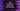# Conditional expression in Dart# Conditional expression in Dart :

Conditional expressions are used in place of if-else statements. These expressions are short, concise and easy to understand.

Two different types of conditional expressions are available in dart. In this post, I will show you both of these expressions with examples.

## Conditional expression definition :

Following are the definitions of the conditional expressions :

``condition ? first_expression : second_expression``

and,

``first_expression ?? second_expression``

### Explanation :

The first expression checks the condition first. If it is true, it executes the firstexpression, else, it executes the _secondexpression_.

If we write the first one using if-else, it looks like as below :

``````if(condition){
return first_expression;
}else{
return second_expression;
}``````

The second expression checks for null of the firstexpression. If it is _null, it executes the secondexpression. _if-else syntax for this expression is like below :

``````if(first_expression != null){
return first_expression;
}else{
return second_expression;
}``````

### Example 1: Find out the larger number using conditional expression :

Let’s write one program to find the larger number using conditional expression. If you use if-else, it will be like below :

``````main() {
int num1 = 10;
int num2 = 9;

int largerNum;

if (num1 > num2) {
largerNum = num1;
} else {
largerNum = num2;
}
print(largerNum);
}``````

Using conditional expression :

``````main() {
int num1 = 10;
int num2 = 9;

int largerNum = num1 > num2 ? num1 : num2;

print(largerNum);
}``````

Both programs will print 10 as the output.

### Example 2 : Check null using conditional expression :

``````main() {
String str1 = null;
String str2 = "str1 is null";

String result = str1 ?? str2;
print(result);
}``````

This example will print “str1 is null”. Because the value of str1 is null. So, it will assign str2 to result.